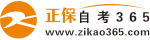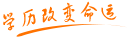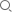#24小时客服：4008135555/010-82335555# 自考“C语言程序设计”模拟试题十

2007年05月29日    来源：   字体：   打印

一、单项选择题（共30分，每题1分）

1. 下列不正确的转义字符是（   ）

A.\\    B.\‘   C.074   D.\0

2. 不是C语言提供的合法关键字是（  ）

A.switch   B.cher   C.case    D.default

3.正确的标识符是（    ）

A.？a   B.a=2   C.a.3   D.a_3

4.下列字符中属于键盘符号的是（  ）

A.\   B.\n   C.\t  D.\b

5.下列数据中属于“字符串常量”的是（   ）

A.ABC   B.“ABC”   C.‘ABC’  D.‘A’

6.char型常量在内存中存放的是（  ）

A.ASCII码   B.BCD码   C.内码值   D.十进制代码值

7.设a为5，执行下列语句后，b的值不为2的是（  ）

A.b=a/2    B.b=6-（——a）  C.b=a%2   D.b=a>3？2：2

8.在以下一组运算符中，优先级更高的运算符是（  ）

A.<=   B.=    C.%    D.&&

9.设整型变量i的值为3，则计算表达式i——i后表达式的值是（）

A.0   B.1    C.   2   D.表达式出错

10.设整型变量a，b，c均为2，表达式a+++b+++c++的结果是（   ）

A.6    B.9    C.8   D.表达式出错

11.若已定义x和y为double类型，则表达式x=1，y=x+3/2的值是（  ）

A.1     B.2    C.2.0    D.2.5

12.设a=1，b=2，c=3，d=4，则表达式：a<b？a：c<d？a：d的结果是（  ）

A.4   B.3   C.2   D.1

13.设a为整型变量，不能正确表达数学关系：10<a<15的C语言表达式是（）

A.10<a<15       B.a= =11 || a= =12 || a= =13 || a= =14

C.a>10&&a<15   D.！（a<=10）&&！（a>=15）

14.若有以下定义：char a、 int b 、 float c 、 double d，则表达式a*b+d-c值的类型为（）

A.float   B.int   C.char   D.double

15.表达式“10！=9”的值是（   ）

A.true   B.非零值   C.0    D.1

16.循环语句 for （x=0，y=0； （y！=123）|| （x<4）；x++）；的循环执行（  ）

A.无限次   B.不确定次   C.4次   D.3次

17.在C语言中，下列说法中正确的是（    ）

A. 不能使用“do while”的循环

B.“do while”的循环必须使用break语句退出循环

C.“do while”的循环中，当条件为非0时将结束循环

D.“do while”的循环中，当条件为0时将结束循环。

18.设a，b为字符型变量，执行scanf（“a=%c，b=%c”，&a，&b）后使a为‘A’，b为‘B’，从键盘上的正确输入是（   ）

A.‘A’ ‘B’    B.‘A’，‘B’    C.A=A，B=B    D.a=A，b=B

19.设i，j，k均为int型变量，执行完下面的for循环后，k的值为（  ）

for （i=0，j=10；i<=j；i++，j——）k=i+j；

A.10    B.9    C.8   D.7

20.设有定义：char s={“string”}；则printf （“%d\n”，strlen（s））；的输出是（  ）

A.6    B.7   C.11    D.12

21.合法的数组定义是（）

A.int a[]={“string”}；   B.int a={0，1，2，3，4，5}；

C.char a={“string”}；   D.char a[]={0，1，2，3，4，5}

22.函数调用strcat（strcpy（str1，str2），str3）的功能是（）

A. 将字符串str2复制到字符串str1中后再将字符串str3连接到字符串str1之后

B. 将字符串str1复制到字符串str2中后再连接到字符串str3之后

C. 将字符串str1复制到字符串str2中后再复制到字符串str3之后

D. 将字符串str2连接到字符串str1中后再将字符串str1复制到字符串str3中

23.设有如下定义，则正确的叙述为（   ）

char     x[]={“abcdefg”}；

char     y[]={‘a’，‘b’，‘c’，‘d’，‘e’，‘f’，‘g’}；

A.数组x和数组y等价

B.数组x和数组y的长度相同

C.数组x的长度大于数组y的长度

D.数组x的长度小于数组y的长度

24.设有单精度变量f，其值为13.8.执行语句“n=（（int）f）%3；”后，n的值是（）

A.4     B.1    C.4.333333    D.4.6

25.执行语句“f=（3.0，4.0，5.0），（2.0，1.0，0.0）；”后，单精度变量f的值是（   ）

A.3.0    B.5.0     C.2.0    D.0.0

26.设a、b均为整型变量，下列表达式中不是赋值表达式的是（   ）

A.a=3，4，5    B.a=3*2    C.a=a&&b    D.a=b=4

27.表达式“1？（0？3：2）： （10？1：0）”的值为（   ）

A.3    B.2    C.1   D.0

28.sizeof（double）的结果值是（    ）

A.8    B.4    C.2   D.出错

29.指出下面正确的输入语句是（    ）

A.scanf （“a=b=%d”，&a，&b）；     B.scanf（“a=%d，b=%f”，&m，&f）；

C.scanf（“%3c”，c）；              D.scanf（“%5.2f，&f）；

30.若有以下定义和语句，且0<=i<5，则（     ）是对数组元素地址的正确表示

int a[]={1，2，3，4，5}，*p，i；

p=a；

A.&（a+i）     B.&p     C.a++      D.&p[i]

二、填空（20分，每空1分）

1.在内存中存储“A”要占用（     ）个字节，存储‘A’要占用（     ）字节。

2.符号常量的定义方法是（      ）。

3.能表述“20<x<30或x<-100”的C语言表达式是（     ）。

4.结构化程序设计方法规定程序或程序段的结构有三种：顺序结构、（     ）和（      ）。

5.C语言共提供了三种用于实现循环结构的流程控制语句，它们是（    ）、（   ）和（    ）。

6.若在程序中用到“putchar”，应在程序开头写上包含命令（      ），若在程序中用到“strlen（）”函数时，应在程序开头写上包含命令（      ）。

7.设有定义语句“static int a={{1}，{2}，{3}}”，则a值为（      ），a的值为（      ）。

8.“*”称为（       ）运算符，“&”称为（     ）运算符。

9.赋值表达式和赋值语句的区别在于有无（       ）号。

10.用{}把一些语句括起来称为（      ）语句

11.设a=12、b=24、c=36，对于“scanf （”a=%d，b=%d，c=%d“，&a，&b，&c）；”，输入形式应为（    ）

12.表达式“sqrt（s*（s-a）*（s-b）*（s-c））；”对应的数学式子为（     ）

13.C语言编绎系统在判断一个量是否为“真”时，以0代表“假”，以（     ）代表“真”

三、读程序（30分）

1. main（）

{ int a=10，b=4，c=3；

if （a<b） a=b；

if （a<c） a=c；

printf（“%d，%d，%d”，a，b，c）；}

2. main（ ）

{int  y=9；

for （；y>0；y——）

if （y%3= = 0） {printf （“%d”，——y）；continue；}

3. main（）

{ int x，y；

for （y=1，x=1；y<=50；y++）

{ if （x>=10） break；

if （x%2= =1） { x+=5；continue；}

x-=3；       }

printf （“%d”，y）；}

4. main（）

{ static int a[]={9，7，5，3，1，2，4，6，8}；

int i，j，s1=0，s2=0； i，j，s1=0，s2=0；

for （i=0；i<3；i++）

for （j=0；j<3；j++）

{ if （i= =j ）s1=s1+a[i][j]；

if （i+j= =2） s2=s2+a[i][j]；

}

printf （“%d\n%d\n”，s1，s2）；}

5. main（）

{static char a[]={‘*’，‘*’，‘*’，‘*’，‘*’}；

int i，j，k；

for （i=0；i<5；i++）

{printf （“\n”）；

for （j=0；j<i；j++） printf （“%c”，‘  ’）；

for （k=0；k<5；k++） printf （“%c”，a[k]）；        }     }

6. int fac（int n）

{static int f=1；

f=f*n； return（f）；}

main（）

{int i；

for （i=1；i<=5；i++）

printf （“%d！=%d\n”，i，fac（i））；}

四、编写程序（20分）

1. 编一个程序，输入X的值，按下列公式计算并输出Y的值

2. 编程序按下列公式计算e的值（精度为1e-6）

e=1+1/1！+1/2！+1/3！+……1/n！

• 热门课程
• 报名咨询

• 相关问答
• 热门问答

• 购物车
• APP下载扫一扫，立即下载

安卓版本：

苹果版本：

开发者：北京正保自考教育科技有限公司

应用涉及权限：查看权限>>

APP隐私策略：查看政策>>

• 公众号扫一扫，立即关注

• 微信群进群领试题/资料